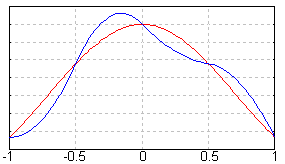# Spline interpolation

In the mathematical subfield of numerical analysis spline interpolation is a special form of interpolation where the interpolant is a piecewise polynomial called spline. Spline interpolation is preferred over polynomial interpolation because the interpolation error can be made small even when using low degree polynomials for the spline. Thus spline interpolation avoids the problem of Runge's phenomenon which occurs when using high degree polynomials.

 Contents

## Definition

Given n+1 distinct knots xi such that

[itex]x_0 < x_1 < ... < x_{n-1} < x_n, \,\! [itex]

with n+1 knot values yi we are trying to find a spline function of degree n

[itex]

S(x) := \left\{\begin{matrix}

   S_0(x) & x \in [x_0, x_1] \\
S_1(x) & x \in [x_1, x_2] \\
\vdots & \vdots \\


S_{k-1}(x) & x \in [x_{k-1}, x_k] \end{matrix}\right. [itex]

with each Si(x) a polynomial of degree n.

## Spline interpolant

Using polynomial interpolation the polynomial of degree n which interpolates the data set is uniquely defined by the data points. The spline of degree n which interpolates the same data set is not uniquely defined and we have to fill in n-1 additional degrees of freedom to construct a unique spline interpolant.

## Linear spline interpolation

Linear spline interpolation is the most simple spline interpolation. Graphically we just connect the data points by straight lines. The spline is just a polygon.

Algebraically each Si is a linear function constructed as

[itex]S_i(x) = y_i + \frac{y_{i+1}-y_i}{x_{i+1}-x_i}(x-x_i)[itex]

The spline must be continuous at each data point, that is

[itex]S_i(x_i) = S_{i+1}(x_i) \qquad \mbox{ , } i=0,\ldots k -1[itex]

This is the case as we can easily see

[itex]S_{i-1}(x_i) = y_{i-1} + \frac{y_{i}-y_{i-1}}{x_{i}-x_{i-1}}(x_i-x_{i-1}) = y_i[itex]
[itex]S_{i}(x_i) = y_i + \frac{y_{i+1}-y_i}{x_{i+1}-x_i}(x_i-x_i) = y_i[itex]

The quadratic spline can be constructed as

[itex]

S_i(x) = y_i + z_i(x-x_i) + \frac{z_{i+1}-z_i}{2(x_{i+1}-x_i)}(x-x_i)^2 [itex]

The coefficients can be found by choosing a [itex]z_0[itex] and then using the recurrence relation:

[itex]

z_{i+1} = -z_i + 2 \frac{y_{i+1}-y_i}{x_{i+1}-x_i} [itex]

## Cubic spline interpolation

For a data set {xi} of n+1 points, we can construct a cubic spline with n piecewise cubic polynomials between the data points. If

[itex]S(x)=\left\{\begin{matrix} S_0(x),\ x\in[x_0,x_1] \\ S_1(x),\ x\in[x_1,x_2]\\ \cdots \\ S_{n-1}(x),\ x\in[x_{n-1},x_n]\end{matrix}\right.[itex]

represents the spline function interpolating the function f, we require:

• the interpolating property, S(xi)=f(xi)
• the splines to join up, Si-1(xi) = Si(xi), i=1,...,n-1
• twice continuous differentiable, S'i-1(xi) = S'i(xi) and S''i-1(xi) = S''i(xi), i=1,...,n-1.

For the n cubic polynomials comprising S, this means to determine these polynomials, we need to determine 4n conditions (since for one polynomial of degree three, there are four conditions on choosing the curve). However, the interpolating property gives us n + 1 conditions, and the conditions on the interior data points give us n + 1 − 2 = n − 1 data points each, summing to 4n − 2 conditions. We require two other conditions, and these can be imposed upon the problem for different reasons.

One such choice results in the so-called clamped cubic spline, with

[itex] S'(x_0) = u \,\![itex]
[itex] S'(x_k) = v \,\![itex]

for given values u and v.

Alternately, we can set

[itex]S''(x_0) = S''(x_n) = 0 \,\![itex].

resulting in the natural cubic spline. The natural cubic spline is approximately the same curve as created by the spline device.

Clamped and natural cubic splines yield the least oscillation about f than any other twice continuously differentiable function

Another choice is gives the periodic cubic spline if

[itex] S(x_0) = S(x_n) \,\![itex]
[itex] S'(x_0) = S'(x_n) \,\![itex]
[itex] S''(x_0) = S''(x_n) \,\![itex]

### Interpolation using natural cubic spline

It can be defined as

[itex]

S_i(x) = \frac{z_{i+1} (x-x_i)^3 + z_i (x_{i+1}-x)^3}{6h_i}

      + \left(\frac{y_{i+1}}{h_i} - \frac{h_i}{6} z_{i+1}\right)(x-x_i)
+ \left(\frac{y_{i}}{h_i} - \frac{h_i}{6} z_i\right) (x_{i+1}-x)


[itex]

and

[itex]h_i = x_{i+1} - x_i \,\![itex].

The coefficients can be found by solving this system of equations:

[itex]

\left\{\begin{matrix}

      z_0 = 0 \\

      h_{i-1}            z_{i-1}
+ 2(h_{i-1} + h_i) z_i
+ h_i              z_{i+1}

      = 6 \left(
\frac{y_{i+1}-y_i}{h_i} -
\frac{y_i-y_{i-1}}{h_{i-1}}
\right) \\

      z_n = 0


\end{matrix}\right. [itex]

## Example

### Linear spline interpolation

Consider the problem of finding a linear spline for

[itex]f(x) = e^{-x^2} [itex]

with the following knots

[itex] (x_0,f(x_0)) = (x_0,y_0) = \left(-1,\ e^{-1}\right) \,\! [itex]
[itex] (x_1,f(x_1)) = (x_1,y_1) = \left(-\frac{1}{2},\ e^{-\frac{1}{4}}\right) \,\! [itex]
[itex] (x_2,f(x_2)) = (x_2,y_2) = \left(0,\ 1\right) \,\! [itex]
[itex] (x_3,f(x_3)) = (x_3,y_3) = \left(\frac{1}{2},\ e^{-\frac{1}{4}}\right) \,\! [itex]
[itex] (x_4,f(x_4)) = (x_4,y_4) = \left(1,\ e^{-1}\right) \,\! [itex]

After directly applying the spline formula, we get the following spline:

[itex]

S(x) = \left\{\begin{matrix} e^{-1} + 2(e^{-\frac{1}{4}} - e^{-1})(x+1) & x \in [-1, -\frac{1}{2}] \\ e^{-\frac{1}{4}} + 2(1-e^{-\frac{1}{4}})(x+\frac{1}{2}) & x \in [-\frac{1}{2},0] \\ 1 + 2(e^{-\frac{1}{4}}-1)x & x \in [0,\frac{1}{2}] \\ e^{-\frac{1}{4}} + 2(e^{-1} - e^{-\frac{1}{4}})(x-\frac{1}{2}) & x \in [\frac{1}{2},1] \\ \end{matrix}\right. [itex]

The spline function (blue lines) and the function it is approximating (red dots) are graphed below:

Missing image
Linearspline.png

The graph below is an example of a spline function (blue lines) and the function it is approximating (red lines) for k=4:• Art and Cultures
• Countries of the World (http://www.academickids.com/encyclopedia/index.php/Countries)
• Space and Astronomy